Newton’s Law of Gravitation

• Last Updated : 20 May, 2021

While an apple could not have fallen on Sir Isaac Newton’s head as history has it, it did inspire him to make one of the most important discoveries in mechanics: the Law of Universal Gravitation. Newton discovered that the Earth itself would be responsible for the apple’s downward motion after pondering why it never falls downwards, backward, or in any other direction but perpendicular to the ground. Newton was able to formulate a general physical law by deduction after hypothesizing that this force would be equal to the masses of the two forces concerned by using existing observations regarding the inverse-square relationship of the force between the earth and the moon. Therefore, let’s first discuss this gravitational force.

What is the Gravitational Force?

Gravitational force is the force of attraction between any two bodies. All the objects in the universe attract each other with a certain amount of force, but in most cases, the force is too weak to be observed due to the very large distance of separation. This force of attraction, also known as Gravitational Force was first identified by a great scientist named Isaac Newton. This concept was developed as Newton’s Universal law of Gravitation in the year 1680.

Important Properties of the gravitational force are:

• Gravitational force is the weakest force in nature but has infinite range.
• Gravitational force is always attractive.
• Gravitational force acts between any two pieces of matter in the Universe since mass is its source.
• Gravitational force is a central force that depends only on the position of test mass from the source mass.
• Gravitational force always acts along the line joining the centers of two bodies.

Some examples of the gravitational force are:

1. If someone jumps upwards in the sky, someone can’t float in the air, it again comes back to earth due to gravitational force.
2. If a ball is thrown upwards, it reaches a certain height and falls downwards because of gravitational force.
3. The force of attraction between the sun and the planets in the Universe is Gravitational Force.

Newton’s Law of Gravitation

According to the Law of Universal Gravitation, every point mass in the universe draws every other point mass in the universe by a force pointing in a straight line between their centers-of-mass, and this force is equal to the masses of the particles and inversely proportional to their difference. From one point to the next, this attractive force often points inward.

This law applies to all objects with weights, no matter how large or small they are. If the difference between two massive objects is very large in comparison to their sizes or if they are spherically symmetric, they may be called point-like masses. In these cases, each object’s mass may be measured by a point mass at its center of mass.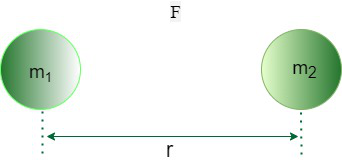Representation of two masses for Newton’s Law of Gravitation

Thus, according to Newton’s law of gravitation, Every particle in the universe attracts every other particle with a force whose magnitude is,

• Directly proportional to the product of their masses i.e.

F ∝ (m1 × m2)                                                                                                                                                                                                     ……(1)

• Inversely proportional to the square of the distance between their centers (Also known as Inverse Square law) i.e.

F ∝ (1 / r2)                                                                                                                                                                                                          ……(2)

On combining equations (1) and (2) it is obtained that,

F ∝ (m1 × m2) / r2                                                                                                                                                                                              ……(3)

Therefore, from equation (3), it can be said that the gravitational force or force of gravity between two particles or objects is proportional to the product of their masses and the square of the distance between them.

F = G × (m1 × m2) / r2                                                                                                                                                                                       ……(4)

Here G is the proportionality constant, also known as Gravitational constant which is always a universal constant,

F is the force of gravity,

m1 is the mass of one object,

m2 is the mass of the other object and

r is the distance between those objects.

What is the Gravitational Constant?

Gravitational Constant is a proportionality constant, and it is used in Newton’s Universal Law of Gravitation. It is denoted by G.

It can also be defined as a force of attraction between any two unit masses separated by unit distance. From Newton’s Universal Law of Gravitation i.e. from equation (4), the expression for G is:

F = G × (m1 × m2) / r2

G = F × r2 / (m1 × m2)

When r =1 m and m1 = m2 = 1 kg. then

G = F

Dimensional Formula for G is [M-1L3T-2].

Its values in SI units is 6.67 × 10-11 Nm2 kg-2 and in CGS system is 6.67×10-8 dyne cm2 g-2.

Importance of Gravitational Law

The universal law of Gravitation successfully explained many concepts like:

• How the planetary motion takes place?
• How all the objects in the Universe affect each other?
• How the lunar motion around the earth takes place?
• How we always kept on the ground and how gravity is responsible for the weight of the body?

Vector Form of Newton’s Law of Gravitation

The action-reaction pair formed by the gravitational forces acting between the two particles is called the vector form of Newton’s law of gravitation. Consider two point mass bodies A and B of masses m₁ and m₂ placed at distance r apart as shown in the below figure: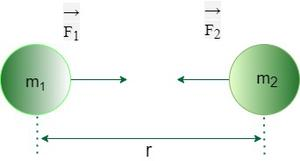Here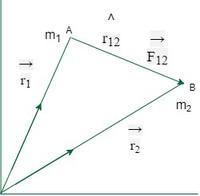From the above figure, it can be seen that the two particles of masses and are placed at a distanceTherefore, according to Newton’s law of gravitation, the force applied on m2 by m1 isSimilarly, Gravitational force on m1 by m2We know that⇒ | r12 |2 = | r21 |2

Thus, it is obtained that:This implies that the above two vectors are equal and opposite to each other which says that gravitational force satisfies Newton’s third law.

Hence, this vector form of Newton’s law of gravitation signifies that the gravitational forces acting between the two particles form the action-reaction pair as shown above.

Principle of Superposition of Gravitational Forces

The principle of the superposition of gravitational forces states that total gravitational force on any object is equal to the sum of individual gravitational forces acting on that object. In other words, the net gravitational field at a point is the vector sum of the gravitational fields at that point due to different sources and is given as:whereis the total gravitational force on an object,andare independent forces acting separately on that object.

Deduction of Newton’s Law of Gravitation from Kepler’s Law

Suppose a planet of mass m is revolving around the sun of mass M in a nearly circular orbit of radius r, with a constant angular velocity ω. Let T be the time period of revolution of the planet around the sun.

Therefore,

F = mrω2

= mr × (2π/T)2                                                                                                                                                                                                    ……(1)

According to Kepler’s third law, the square of the time period of the planet is proportional to squares of length of semi-major axes and is given as:

T2 ∝ r3

or

T2 = kr3

where k is the proportionality constant, T is the time period of the planet and r is the length of the semi-major axis.

Now apply this in the equation (1) as:

F = mr × (4π2 / kr3)

= (4π2 × m) / kr2

But k = 4π2 / GM, substitute this in the above expression as:

F = (G × M × m) / r2

which is the equation of Newton’s law of Gravitation.

Hence, Newton deduced the following from Kepler’s Laws:

1. A force occurring on the earth due to the sun is the centripetal force, which is oriented towards the sun.
2. The planet’s gravity must be inversely proportional to the square of its distance from the sun.
3. The force operating on the earth is proportional to the product of the earth’s and sun’s masses.

Sample Problems on the Newton’s Law of Gravitation

Problem 1: Will the gravitational force of attraction be different if the same bodies are taken on to the moon keeping their distance of separation remaining constant?

Solution:

If the same bodies are taken on to the moon keeping their separation distance constant then the gravitational force of attraction between the two bodies remains the same since the gravitational force of attraction between two bodies is unaffected by the presence of the third body and the medium between the two bodies.

Thus, the gravitational force of attraction remains the same between those bodies after taking to the moon.

Problem 2: Two bodies of masses 5 kg and 6 kg are placed with their centers 64 m apart. Calculate the initial acceleration of two masses assuming no other forces act on them.

Solution:

Given that,

The mass of the first body, m1 is 5 kg.

The mass of the second body, m2 is 6 kg.

The distance between the two bodies, r is 64 m.

And the value of universal gravitational constant, G is 6.67 × 10-11 Nm2 kg-2

By the Newton’s law of Gravitation, the formula for gravitational force is given as

F = G × (m1 × m2) / r2

Substitute the given values in the above expression as:

F = 6.67 × 10-11 Nm2 kg-2 × (5 kg × 6 kg) / (64 m)2

= 48.85 × 10-14 N

Now, it is known that, the net force on an object is given by:

F = ma

or

a = F / m

Substitute the given and the obtained values in the above expression to calculate a for two bodies as:

a1 = 48.85 × 10-14 N / 5 kg

= 9.77 × 10-14 m/s2

And

a2 = 48.85 × 10-14 N / 6 kg

= 8.142 × 10-14 m/s2

Thus, the initial accelerations of two bodies are 9.77 × 10-14 m/s2 and 8.142 × 10-14 m/s2 respectively.

Problem 3: A body of mass 1 kg and another body of mass 10 kg are separated by a distance of 100 m, and they are attracted by a force of 19.6×10-10 N. Calculate the value of the universal gravitation constant for the given case.

Solution:

Given that,

The mass of the first body, m1 is 1 kg.

The mass of the second body, m2 is 10 kg.

The distance between the two bodies, r is 100 m.

The gravitational force of attraction between the two bodies F is 19.6 × 10-10 N.

Therefore, from the Newton’s law of Gravitation:

G = F × r2 / (m1 × m2)

= 19.6 × 10-10 N × (100 m)2 / (1 kg × 100 kg)

= 19.6 × 10-7 Nm2 kg-2

Thus, the value of universal gravitation constant for this case is 19.6 × 10-7 Nm2 kg-2.

Problem 4: If the two objects attract each other with a gravitational force of F units. If the mass of both objects was tripled and the distance between the objects also doubled. What would be the new force of attraction between two objects?

Solution:

Let the force of attraction between two bodies be F N.

And it is known from Newton’s law of Gravitation that the force of gravitational attraction is,

F = G × (m1 × m2) / r2

where m1 is the mass of first object, m2 is the mass of second object and r is the distance between the two bodies.

Let F1 be the new force of attraction between two objects.

When the mass of both objects are tripled and the distance between the objects is doubled then the force acting between two objects is,

F1 = G × (3 × m1 × 3 × m2) / (2r)2

= 9G × m1 × m2 / 4r2

= 9F / 4

Thus, the new force of attraction between two objects is 9F / 4 units.

Problem 5: Calculate the resultant force acting on mass m which is at position 1 and all the masses which are equal to m are placed in the vertices of the equilateral triangle having the length of its side is r.

Solution:

Given that, the masses whose weight equal to m are placed on the three corners of equilateral triangle.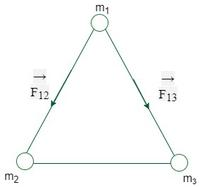Let F1 be the force acting on mass m which is at position 1.

By the Principle of Superposition of Gravitational Forces,F1=forces acting on mass m due to another mass m which is at position 2+forces acting on mass m due to another mass m which is at position 3.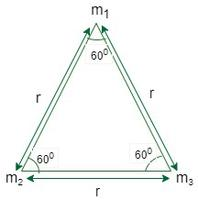Hereandmakes an angle of 60° to each other since they act along the sides of equilateral triangle.LetFres==Fres=√3f=√3×Gm2/r2

Thus the resultant force acting on mass m which is at position 1 and all the masses which are equal to m are placed in the vertices of the equilateral triangle having the length of its side is √3Gm2/r2.

Problem 6: Three uniform spheres each having a mass M and radius a are kept in such a way that each touches the other two.Find the magnitude of the gravitational force on any of the sphere due to the other two spheres.

Solution:

Let A, B and C be the centers of three uniform spheres.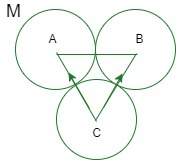Letbe the Force acting on C due to A andbe the force acting on C due to B

Let the total force acting on C due to A and B beBy the Principle of Superposition of Gravitational Forces,

⇒……(1)

On joining A,B and C, we get a equilateral triangle i.e, it makes an angle of 60° to each other.

Given radius of each sphere is a

so, AB=BC=CA =2a

From Newton’s law of Gravitation,HereFrom (1),==|F| =Thus the magnitude of the gravitational force on any of the sphere due to the other two spheres isProblem 7: If mercury, Venus, and the Sun are aligned in a right-angled triangle. Calculate the vector sum of forces on venus due to both mercury and Sun. What is the direction and magnitude of the resulting force?

Solution: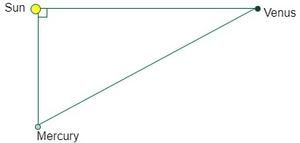Given that,

Distance between Sun and Venus (rv)=108×109 meters.

Distance between Sun and Mercury(rm)=57.6×109 meters

Distance between Mercury and Venus (rmv)=⇒ rmv=1.08×1011 meters.

Mass of Sun= 1.99×1030 kg

Mass of Mercury =3.3×1023 kg

Mass of Venus =4.87×1024 kg

Let Force acting on Venus due to Sun is FS

From Newton’s law of Gravitation,= 6.67×10-11×1.99×1030×4.87×1024/(108×109)^2

FS=5.54×1022

Let Force acting on Venus due to Mercury is FM

⇒= 6.67×10-11×3.3×1023×4.87×1024/(1.08×1011)2

FM= 9.19×1015 N

Here Force due to Sun is more than a million times greater than Force due to Mercury and so the net Force is mainly due to Sun and its magnitude is equal to Force acting due to Sun. And the direction of resulting Force similar to direction due to Sun.

My Personal Notes arrow_drop_up